# Semi-normal operator

(diff) ← Older revision | Latest revision (diff) | Newer revision → (diff)

A bounded linear operator, acting on a Hilbert space, with the property that its self-commutator is trace-class, i.e.. A semi-normal operator can equivalently be defined by a pair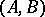of self-adjoint operators (cf. Self-adjoint operator) with trace-class commutator (after writing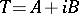). The theory of semi-normal operators is one of the few well-developed spectral theories for a class of non-self-adjoint operators. For the latter, see [a3].

Most examples of semi-normal operators are obtained via the Berger–Shaw inequality: Ifis a hyponormal operator (i.e.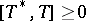), of finite rational cyclicity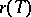, then: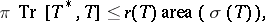whereis the spectrum of(cf. also Spectrum of an operator).

Here, the rational cyclicity(also called the rational multiplicity) of an operatoris defined as follows. Let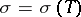be the spectrum ofand let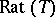be the algebra of rational functions of a complex variable with poles outside. Thenis the smallest cardinal number such that there is a set of vectors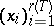such that the closure of the span ofis the whole space.

If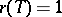, thenis said to be rationally cyclic. In particular, normal and rationally cyclic subnormal operators are semi-normal, [a2]. Certain singular integral operators with Cauchy-type kernel are also semi-normal, see [a3], [a4], [a6] and Singular integral.

One of the most refined unitary invariants of a pure semi-normal operatoris the principal function, which was introduced by J.D. Pincus [a6]. Let,be polynomials in two complex variables, and letbe the Jacobian of the pair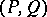, written in complex coordinates. The Pincus–Helton–Howe trace formula [a4] characterizes: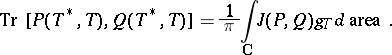Via this formula one can prove the functoriality of the principal function under the holomorphic functional calculus. An observation due to D. Voiculescu shows that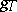is invariant under Hilbert–Schmidt perturbations of. The entire behaviour of the principal function qualifies it as the correct two-dimensional analogue of Krein's spectral shift function, well known in the perturbation theory of self-adjoint operators, see [a5]. The above trace formula can be interpreted as a generalized index theorem; this was one of the origins cyclic cohomology.

Thanks to a deep result of T. Kato and C.R. Putnam, a pure hyponormal operatorwith trace-class self-commutator has absolutely continuous real and imaginary partsand. Consequently, by diagonalizingon a vector-valued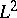-spacesupported on the real line, the operatorbecomes: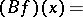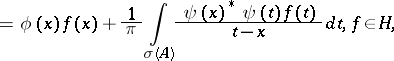where,are essentially bounded operator-valued functions. This singular integral model extends to all semi-normal operators; it was the source of most results in this area, by putting together methods of scattering theory (cf. also Scattering matrix) and singular integral equations, cf. [a1], [a7].

Several invariant-subspace results are known for semi-normal operators. For instance, S.W. Brown has shown that hyponormal operators with thick spectrum (that is, dominant spectrum in an open subset of) have non-trivial invariant subspaces. As an application of the theory of the principal function, C.A. Berger has proved that sufficiently high powers of hyponormal operators have invariant subspaces. For both results see [a5].

One of the most studied sets of semi-normal operators is the class of operatorswith rank-one self-commutator: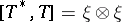. For irreducible, the determinantal function [a6]: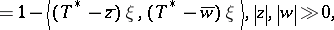is a complete unitary invariant of. This function can be expressed as the exponential of a double Cauchy transform of the principal function. A variety of applications of the above determinantal function to inverse problems of potential theory in the plane are known, [a1], [a5].

How to Cite This Entry:
Semi-normal operator. Encyclopedia of Mathematics. URL: http://encyclopediaofmath.org/index.php?title=Semi-normal_operator&oldid=19046
This article was adapted from an original article by M. Putinar (originator), which appeared in Encyclopedia of Mathematics - ISBN 1402006098. See original article